September 29, 2023

# Relationship between Zeros and coefficients of a Polynomial

Relationship between Zeros and coefficients of a Polynomial

zeros of a polynomial : A real number is called a zero of the polynomial

, if .

If “” is a zero of a polynomial  , then by factor theorem  is  a factor of a given polynomial. The relation between the zeros and the coefficients of a polynomial is given below.

Linear Polynomial:

The linear polynomial is an expression , in which the degree of the polynomial is 1 . The general form of a linear polynomial is  . Here,  is a variable, “a” and “bare constant.

Let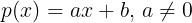be a linear polynomial,

then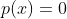means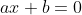.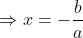So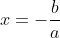=.

Quadratic polynomial:A polynomial of degree 2 is called a quadratic polynomial. A quadratic polynomial in one variable will have at most tree terms.  Any quadratic polynomial in will be of the form

Let and be the zeros of the quadratic polynomial .

Then and are factors of ., where is a constant.

On comparing  coefficients of like powers of on both sides, we get

sum of zeros =    –

product of zeros =

Cubic polynomial :   A polynomial of  degree  3 is called  cubic polynomials. Any  cubic  polynomial can have at  most 4 terms.  Cubic polynomial can be written in the form    and and are constants.

Let ,    and be the zeros of the cubic  polynomial .

Then   and are factors of .

, for some constant .

=

On comparing  coefficients of like powers of on both sides, we get

If ,    and be the zeros of the cubic  polynomial , then

(i)           (ii)          (iii)

Similarly, If α , β, γ, δ are roots of the  equation , then

.

Some practice questions based on polynomial are given in the following worksheet.#### Bina singh

View all posts by Bina singh →
ssc chsl 2023 tier 1 cut off NIRF Rankings 2023 : Top 10 Engineering colleges in India CBSE Compartment Exam 2023 Application Form SSC CGL 2023 Notification: Important Dates UPSC Recruitment 2023